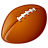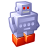# Summing up the length of edges

708
7
05-24-2012 07:16 AMNew Contributor II
Hi,

I´m currently diving into CGA scripts. I´ve checked out this forum, read some articles in the documentation and worked through several examples. Still some aspects of how CE evaluates rules remain a mystery to me. As a start I want to calculate the length of all edges at the side of a street. I tried the following code but the attributes are never set (the edge indices are correct though):
```attr streetSideLengthMin = 0
attr streetSideLengthMax = 0

Street -->
SetupStreetAttributes
print("After SetupStreetAttributes")

<more code following here>

SetupStreetAttributes -->
[comp(e){ all : calc}]

calc -->
case comp.index == 0 || ((comp.index > 1) && ((comp.index - 1) % 3 == 0)):
print("Before: " + streetSideLengthMin)
set( streetSideLengthMin, streetSideLengthMin + scope.sx)
print("After: " + streetSideLengthMin)
else:
case comp.index == 2 || ((comp.index > 3) && (comp.index % 3 == 0)):
set( streetSideLengthMax, streetSideLengthMax + scope.sx)
else:
NIL
```

Probably I am completely misusing the rules here... ;)

My goal is to initially calculate the two attributes and then go on executing the shape generation process based on the attribute values. I decided to use rules instead of functions because of the comp operator which actually comes in quiet handy here. Using "print" messages for debugging indicated that the execution order is not as expected e.g. like in usual programming languages. Even the print statement after "SetupStreetAttributes" seems to print to console before "SetupStreetAttributes" is actually executed.

I would be happy to understand why this approach doesn´t work and how the task could be achieved correctly. If anybody can point me to the appropriate docs I would be happy to go through it.

Regards,
Mirko
Tags (3)
7 RepliesNew Contributor II
As far as I understand the execution of rules follows a strong hierarchical order where the uppermost rule is fully executed first before "child" rules are executed (independent of the call-order). Is this a correct interpretation? I did not find it in the docs yet.

I´ve checked a previous example you gave here. There you use a recursive algorithm leading to the correct execution of "child" rules. Following my approach I´m wondering if it is actually possible to calculate and globally store values e.g. in attributes using convenience functions like "comp" etc. ...

Regards,
MirkoFrequent Contributor II
Hi .

Rules pass the shapes down to the next rules. Due to that 'passing on', previous data cannot be accessed unless it is passed explicitly.

The access to geometric values is currently still limited in CityEngine, thus the only way to compute the sum of the edge lengths would need to be done with a complex recursive rule. I've written such a thing once to find the index of the shortest edge (or so).

There are still some limitations, but we're working hard to improve such details.New Contributor II
Hi Matthias,

So is there a way to set attributes initially as described above? Since its not possible (as fas as I understand) to use geometric operations like "comp" within functions (not rules) how would an iterative algorithm work?

We are looking forward developing more complex CGA algorithms. Can you provide some hints in which direction CGA will develop in upcoming releases?

Regards,
MirkoFrequent Contributor II
Passing values on :

```RuleA -->
RuleB(5)

RuleB(myVal) -->
print (myVal)
```

or :

```attr myValue = 0 # initialize attr

RuleA -->
set (myValue , 15)
RuleB

RuleB -->
print (myValue)
```

* * *

About CGA development : Cannot really share :)

* * *

a simple recursive rule :

```attr startValue = 20

Lot -->
Recursion(startValue)

Recursion(n) -->
case n > 0 : # break !
Printer(n)
Recursion(n - 1)
else :
NIL

Printer(n) -->
print (n)
```New Contributor II
Hi Matthias,

Thank you for the examples. I set global attributes in my example code in the first post but did not succeed since the execution order was different from what I expected. I would like to follow the recursive approach you sketch in your last post and the previous example to mark corner lots here.

My question is:
Is it possible to define a recursive rule which accesses individual edges of the base shape (to e.g. query their length using scope.sx), store the calculated values and finally call a rule which performs operations on the same (unmodified) base shape?

I don´t see a way to access components of a shape, calculate some values based on them, and resume calling a rule with the current shape. It seems that the logic is limited to a top-down order...

Regards,
MirkoFrequent Contributor II
Hi ..

to 'properly' access geometric values such as 'geometry.area()', we have some operations, but that list could be extended forever.

CGA is at the moment, as you state correctly, limited to the top-down order. Thus, if you e.g. split a shape into 2 separate child shapes, they have no more knowledge of the existence of the each other. An other thing, if you split a shape, it may happen that depending on the topology of a shapes, 2, 3 or more children are created (concave shape). How many will be resulting is not possible to query prior to actually splitting.

the scope always encompasses the whole shape geometry, thus the edge length is only then correct if it's a planar rectangle you're dealing with. otherwise, you need a different approach, if this is the goal.

Here's the code to find the longest edge :
Again, this is total overkill and shall not be a sample of proper code !

What I do here is do some vector math, calculating the distanced from 1 reported vertex position to the next, comparing the index of the longest edge until the recursion is done.

```version "2011.2"

@Hidden
attr currentLongestEdgeID = 0
@Hidden
attr currentLongestEdgeLength = 0

@Hidden
attr startPivotPosX = 0
@Hidden
attr startPivotPosY = 0
@Hidden
attr startPivotPosZ = 0

@Hidden
attr endPivotPosX = 0
@Hidden
attr endPivotPosY = 0
@Hidden
attr endPivotPosZ = 0

dist (sX, sY, sZ, eX, eY, eZ) =
# space diagonal
sqrt ( (eX-sX) * (eX-sX) + (eY-sY) * (eY-sY) + (eZ-sZ) * (eZ-sZ) )

FindEdgeIndexOfLongestEdge -->
# align to edge BEFORE first edge
alignScopeToGeometry(yUp, 0, geometry.nVertices)
# set first END point
set (endPivotPosX, convert(x,pivot,world,pos,0,0,0))
set (endPivotPosY, convert(y,pivot,world,pos,0,0,0))
set (endPivotPosZ, convert(z,pivot,world,pos,0,0,0))
EdgeRecursion(0, geometry.nVertices) # check all edges !

EdgeRecursion (current, max) -->
case current <= max :
# main loop over all edge indices

alignScopeToGeometry(yUp, 0, current)
# print (current)
# print (scope.sx)

# new start = old end
set (startPivotPosX, endPivotPosX)
set (startPivotPosY, endPivotPosY)
set (startPivotPosZ, endPivotPosZ)

# new end
set (endPivotPosX, convert(x,pivot,world,pos,0,0,0))
set (endPivotPosY, convert(y,pivot,world,pos,0,0,0))
set (endPivotPosZ, convert(z,pivot,world,pos,0,0,0))

SetAttributes(current, max)
NIL
else :
#bail out
print ("________________________")
print (currentLongestEdgeID)
print (currentLongestEdgeLength)
NIL

SetAttributes (current, max) -->
case dist (startPivotPosX,startPivotPosY,startPivotPosZ,endPivotPosX,endPivotPosY,endPivotPosZ) > currentLongestEdgeLength :
set (currentLongestEdgeLength, dist (startPivotPosX,startPivotPosY,startPivotPosZ,endPivotPosX,endPivotPosY,endPivotPosZ))
set (currentLongestEdgeID, current - 1) # -1 because we calculate a first edge for the correct vector of the first edge !
EdgeRecursion(current + 1, max)
else :
EdgeRecursion(current + 1, max)

```Frequent Contributor II
And here's an other example of recursion :

[ATTACH=CONFIG]14747[/ATTACH]

see attached files.

That's basically the same approach as used for the Abandone Space Station I made quite some time ago :